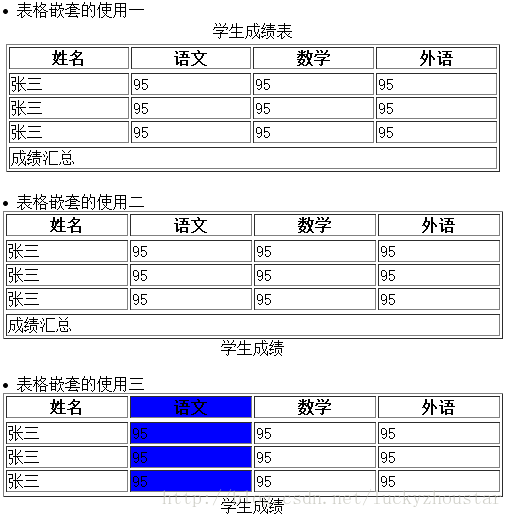﻿ 浅谈Html网页表格结构化标记的应用_移动前端-图趣网（Tuquu）

# 浅谈Html网页表格结构化标记的应用Html表格的结构化

XML/HTML Code复制内容到剪贴板
1. <table>
2.
4.
5. <tbody></tbody>---------表体区
6.
7. <tfoot></tfoot>------------表尾区
8.
9. </table>

Html表格的题目

XML/HTML Code复制内容到剪贴板
1. <table>
2.
3. <caption></caption>
4.
5. </table>
<caption>下的属性值有：

align

Top

Bottom

Html直列化格式

<colgroup>…<colgroup>

Align

Left

Center

Right

Valign

Right

Top

Middle

Bottom

Span

XML/HTML Code复制内容到剪贴板
1. <html>
3.
4. <li>表格嵌套的使用一</li>
5.
6. <table  width="500" >
7. <tr>
8. <td align="center">门生成绩表</td>
9. </tr>
10. <td>
11. <table border="1" width="100%">
13. <tr>
14. <th>姓名</th>
15. <th>语文</th>
16. <th>数学</th>
17. <th>外语</th>
18. </tr>
20. <tbody>
21. <tr>
22. <td>张三</td>
23. <td>95</td>
24. <td>95</td>
25. <td>95</td>
26. </tr>
27. <tr>
28. <td>张三</td>
29. <td>95</td>
30. <td>95</td>
31. <td>95</td>
32. </tr>
33. <tr>
34. <td>张三</td>
35. <td>95</td>
36. <td>95</td>
37. <td>95</td>
38. </tr>
39. </tbody>
40. <tfoot>
41. <tr>
42. <td colspan="4">成绩汇总</td>
43. </tr>
44. </tfoot>
45. </table>
46. </td>
47. </tr>
48. </table>
49.
50. <br/>
51.
52. <li>表格嵌套的使用二</li>
53.
54. <table border="1" width="500" >
55. <caption align="bottom">门生成绩</caption>
57. <tr>
58. <th>姓名</th>
59. <th>语文</th>
60. <th>数学</th>
61. <th>外语</th>
62. </tr>
64. <tbody>
65. <tr>
66. <td>张三</td>
67. <td>95</td>
68. <td>95</td>
69. <td>95</td>
70. </tr>
71. <tr>
72. <td>张三</td>
73. <td>95</td>
74. <td>95</td>
75. <td>95</td>
76. </tr>
77. <tr>
78. <td>张三</td>
79. <td>95</td>
80. <td>95</td>
81. <td>95</td>
82. </tr>
83. </tbody>
84. <tfoot>
85. <tr>
86. <td colspan="4">成绩汇总</td>
87. </tr>
88. </tfoot>
89. </table>
90.
91. <br/>
92.
93. <li>表格嵌套的使用三</li>
94. <table border="1" width="500" >
95. <caption align="bottom">门生成绩</caption>
96. <col ></col>
97. <col bgcolor=blue></col>
99. <tr>
100. <th>姓名</th>
101. <th>语文</th>
102. <th>数学</th>
103. <th>外语</th>
104. </tr>
106. <tbody>
107. <tr >
108. <td>张三</td>
109. <td>95</td>
110. <td>95</td>
111. <td>95</td>
112. </tr>
113. <tr>
114. <td>张三</td>
115. <td>95</td>
116. <td>95</td>
117. <td>95</td>
118. </tr>
119. <tr>
120. <td>张三</td>
121. <td>95</td>
122. <td>95</td>
123. <td>95</td>
124. </tr>
125. </tbody>
126. <tfoot>
127. </tfoot>
128. </table>
129.
130. </body>